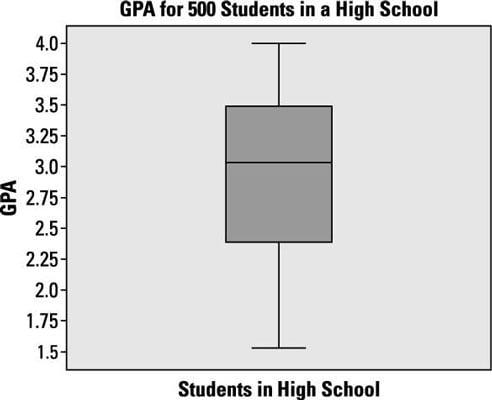##### Statistics: 1,001 Practice Problems For DummiesBox plots are a huge issue. Making a box plot itself is one thing; understanding the do's and (especially) the don'ts of interpreting box plots is a whole other story.

The following box plot represents data on the GPA of 500 students at a high school.Credit: Illustration by Ryan Sneed

## Sample questions

1. What is the range of GPAs in this data?

The range of data is from 1.5 to 4.0, which is 4.0 –d 1.5 = 2.5.

2. What is the median of the GPAs?

The thick line within the box indicates the median (or middle number) for the data.

3. What is the IQR for this data?

The interquartile range (IQR) is the distance between the 1st and 3rd quartiles (Q1 and Q3). In this case, IQR = 3.5 – 2.375 = 1.125.

4. What does the scale of the numerical axis signify in this box plot?

The numerical axis is a scale showing the GPAs of individual students ranging from 1.5 to 4.0.

5. Where is the mean of this data set?

A box plot includes five values: the minimum value, the 25th percentile (Q1), the median, the 75th percentile (Q3), and the maximum value. The value of the mean isn't included on a box plot.

6. What is the approximate shape of the distribution of this data?

You can't tell the exact distribution of data from a box plot. But because the median is located above the center of the box and the lower tail is longer than the upper tail, this data is skewed left.

7. What percentage of students has a GPA that lies outside the actual box part of the box plot?

The actual box part of a box plot includes the middle 50% of the data, so the remaining 50% of the total must be outside the box.

8. What percentage of students has a GPA below the median in this data?

The definition of a median is that half the data in a distribution is below it and half is above it. In a box plot, the median is indicated by the location of the line inside the box part of the box plot.

If you need more practice on this and other topics from your statistics course, visit 1,001 Statistics Practice Problems For Dummies to purchase online access to 1,001 statistics practice problems! We can help you track your performance, see where you need to study, and create customized problem sets to master your stats skills.